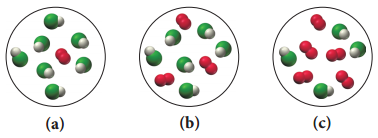# Problem: Consider the reaction:4 HCl(g) + O2(g) → 2 H2O(g) + 2 Cl2(g)Each molecular diagrams represents an initial mixture of the reactants.How many molecules of Cl2 would be formed from the reaction mixture that produces the greatest amount of products?

###### FREE Expert Solution

HCl is oxidized by O2 to produce water and Cl2. We have three different mixtures of reactants and we have to determine which mixture produces the greatest amount of Cl2 gas.

We will solve for the number of molecules of Cl2 from each reactant mixture and determine the limiting reactant in each case.

85% (459 ratings)###### Problem Details
Consider the reaction:

4 HCl(g) + O2(g) → 2 H2O(g) + 2 Cl2(g)

Each molecular diagrams represents an initial mixture of the reactants.How many molecules of Cl2 would be formed from the reaction mixture that produces the greatest amount of products?